• Slides: 94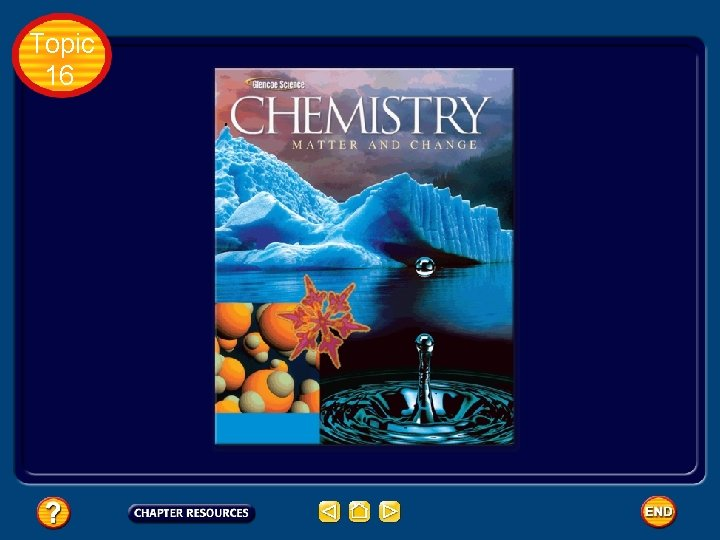Topic 16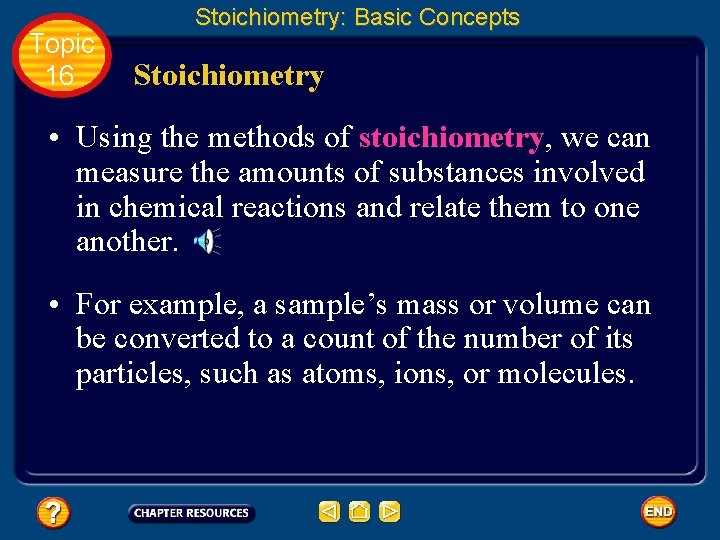Topic 16 Stoichiometry: Basic Concepts Stoichiometry • Using the methods of stoichiometry, we can measure the amounts of substances involved in chemical reactions and relate them to one another. • For example, a sample’s mass or volume can be converted to a count of the number of its particles, such as atoms, ions, or molecules.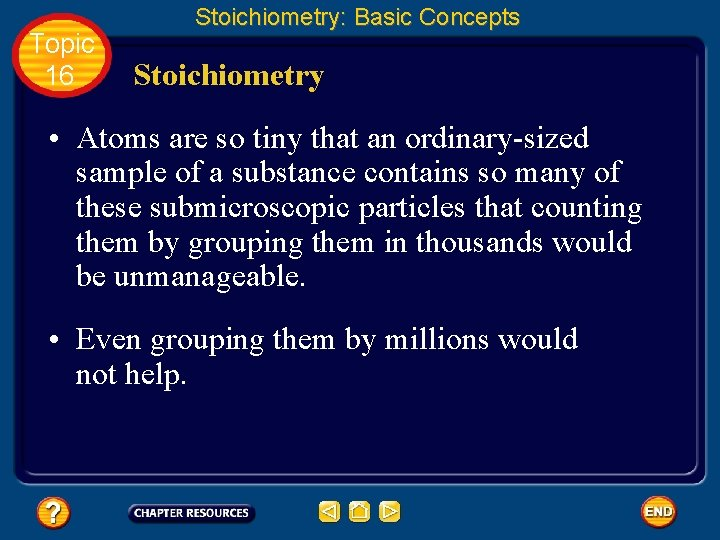Topic 16 Stoichiometry: Basic Concepts Stoichiometry • Atoms are so tiny that an ordinary-sized sample of a substance contains so many of these submicroscopic particles that counting them by grouping them in thousands would be unmanageable. • Even grouping them by millions would not help.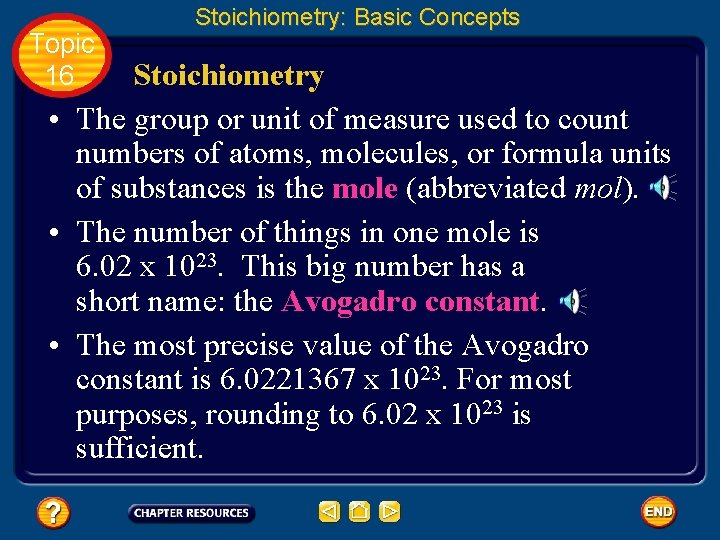Topic 16 Stoichiometry: Basic Concepts Stoichiometry • The group or unit of measure used to count numbers of atoms, molecules, or formula units of substances is the mole (abbreviated mol). • The number of things in one mole is 6. 02 x 1023. This big number has a short name: the Avogadro constant. • The most precise value of the Avogadro constant is 6. 0221367 x 1023. For most purposes, rounding to 6. 02 x 1023 is sufficient.Topic 16 Stoichiometry: Basic Concepts Molar Mass • Methanol is formed from CO 2 gas and hydrogen gas according to the balanced chemical equation below.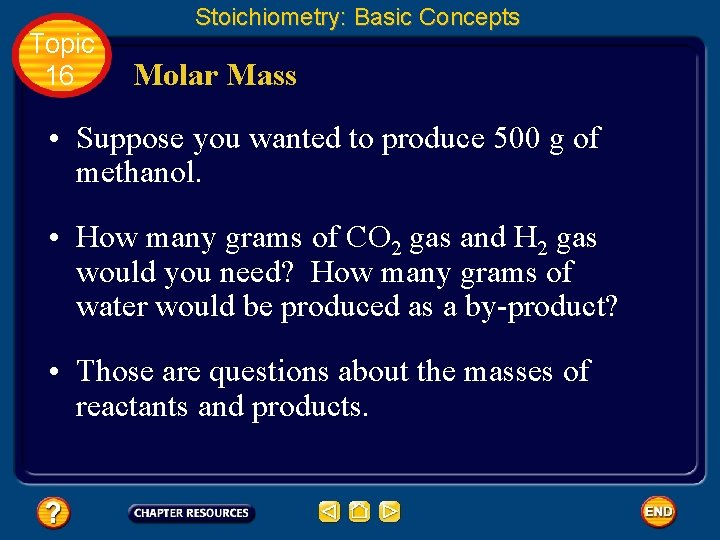Topic 16 Stoichiometry: Basic Concepts Molar Mass • Suppose you wanted to produce 500 g of methanol. • How many grams of CO 2 gas and H 2 gas would you need? How many grams of water would be produced as a by-product? • Those are questions about the masses of reactants and products.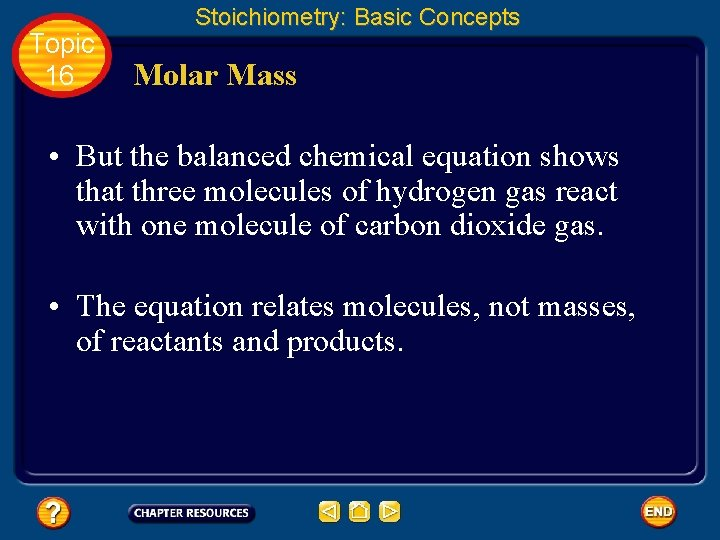Topic 16 Stoichiometry: Basic Concepts Molar Mass • But the balanced chemical equation shows that three molecules of hydrogen gas react with one molecule of carbon dioxide gas. • The equation relates molecules, not masses, of reactants and products.Topic 16 Stoichiometry: Basic Concepts Molar Mass • Like Avogadro, you need to relate the macroscopic measurements—the masses of carbon dioxide and hydrogen—to the number of molecules of methanol. • To find the mass of carbon dioxide and the mass of hydrogen needed to produce 500 g of methanol, you first need to know how many molecules of methanol are in 500 g of methanol.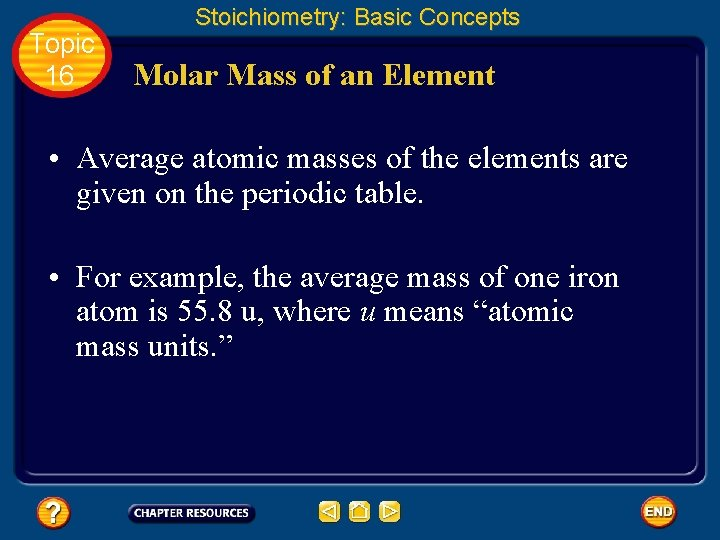Topic 16 Stoichiometry: Basic Concepts Molar Mass of an Element • Average atomic masses of the elements are given on the periodic table. • For example, the average mass of one iron atom is 55. 8 u, where u means “atomic mass units. ”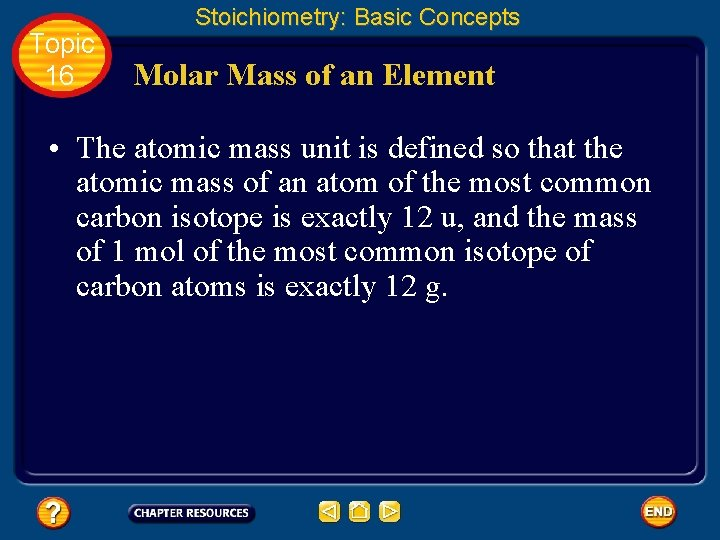Topic 16 Stoichiometry: Basic Concepts Molar Mass of an Element • The atomic mass unit is defined so that the atomic mass of an atom of the most common carbon isotope is exactly 12 u, and the mass of 1 mol of the most common isotope of carbon atoms is exactly 12 g.Topic 16 Stoichiometry: Basic Concepts Molar Mass of an Element • The mass of 1 mol of a pure substance is called its molar mass.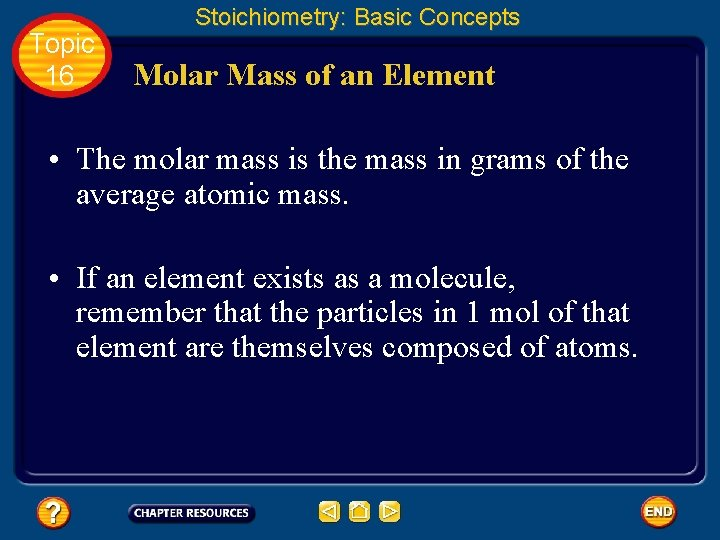Topic 16 Stoichiometry: Basic Concepts Molar Mass of an Element • The molar mass is the mass in grams of the average atomic mass. • If an element exists as a molecule, remember that the particles in 1 mol of that element are themselves composed of atoms.Topic 16 Stoichiometry: Basic Concepts Molar Mass of an Element • For example, the element oxygen exists as molecules composed of two oxygen atoms, so a mole of oxygen molecules contains 2 mol of oxygen atoms. • Therefore, the molar mass of oxygen molecules is twice the molar mass of oxygen atoms: 2 x 16. 00 g = 32. 00 g.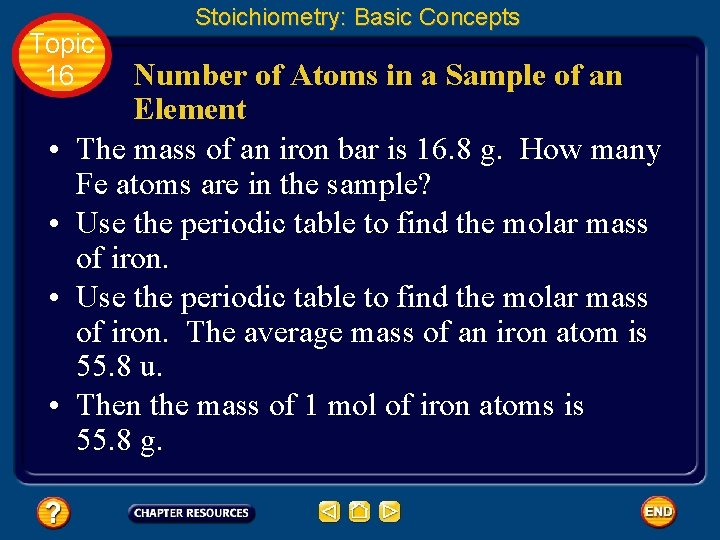Topic 16 • • Stoichiometry: Basic Concepts Number of Atoms in a Sample of an Element The mass of an iron bar is 16. 8 g. How many Fe atoms are in the sample? Use the periodic table to find the molar mass of iron. The average mass of an iron atom is 55. 8 u. Then the mass of 1 mol of iron atoms is 55. 8 g.Topic 16 Stoichiometry: Basic Concepts Number of Atoms in a Sample of an Element • To convert the mass of the iron bar to the number of moles of iron, use the mass of 1 mol of iron atoms as a conversion factor. • Now, use the number of atoms in a mole to find the number of iron atoms in the bar.Topic 16 Stoichiometry: Basic Concepts Number of Atoms in a Sample of an Element • Simplify the expression above.Topic 16 Stoichiometry: Basic Concepts Molar Mass of a Compound • Covalent compounds are composed of molecules, and ionic compounds are composed of formula units. • The molecular mass of a covalent compound is the mass in atomic mass units of one molecule. • Its molar mass is the mass in grams of 1 mol of its molecules.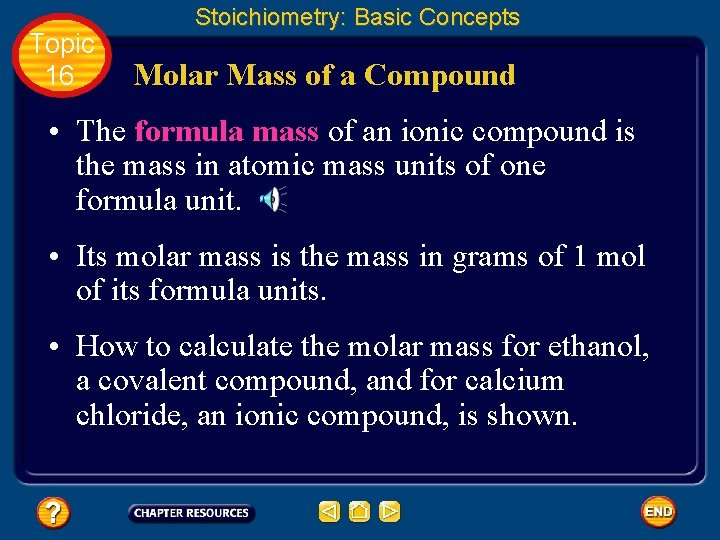Topic 16 Stoichiometry: Basic Concepts Molar Mass of a Compound • The formula mass of an ionic compound is the mass in atomic mass units of one formula unit. • Its molar mass is the mass in grams of 1 mol of its formula units. • How to calculate the molar mass for ethanol, a covalent compound, and for calcium chloride, an ionic compound, is shown.Topic 16 Stoichiometry: Basic Concepts Molar Mass of a Compound • Ethanol, C 2 H 6 O, a covalent compound.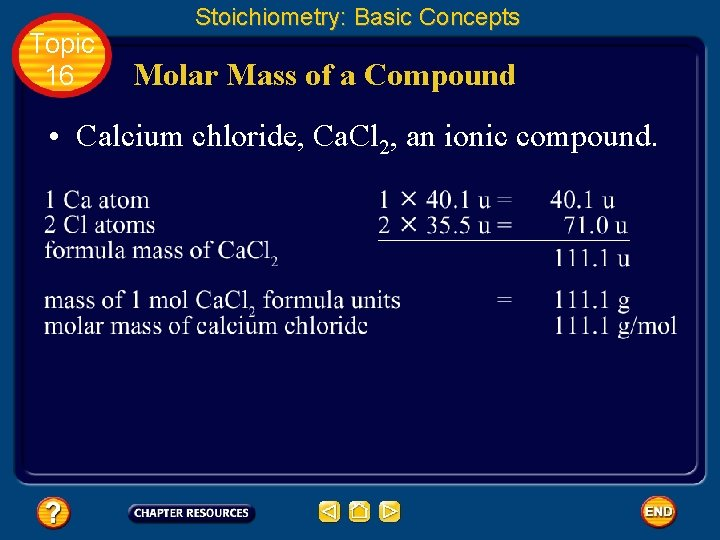Topic 16 Stoichiometry: Basic Concepts Molar Mass of a Compound • Calcium chloride, Ca. Cl 2, an ionic compound.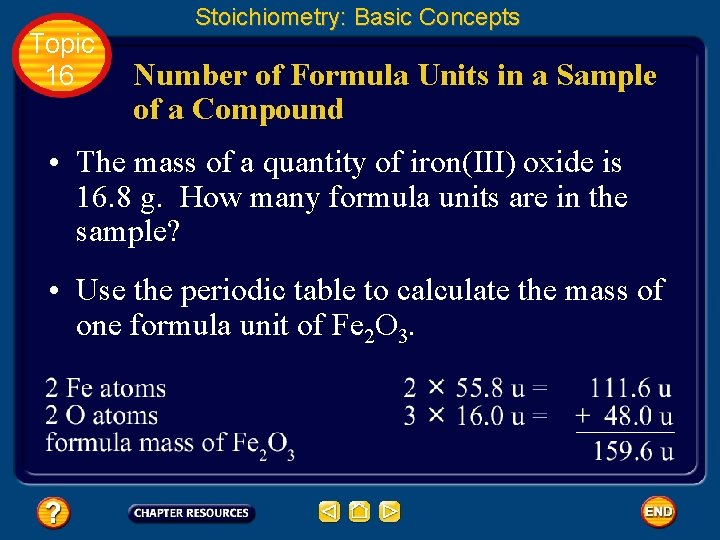Topic 16 Stoichiometry: Basic Concepts Number of Formula Units in a Sample of a Compound • The mass of a quantity of iron(III) oxide is 16. 8 g. How many formula units are in the sample? • Use the periodic table to calculate the mass of one formula unit of Fe 2 O 3.Topic 16 Stoichiometry: Basic Concepts Number of Formula Units in a Sample of a Compound • Therefore, the molar mass of Fe 2 O 3 (rounded off) is 160 g.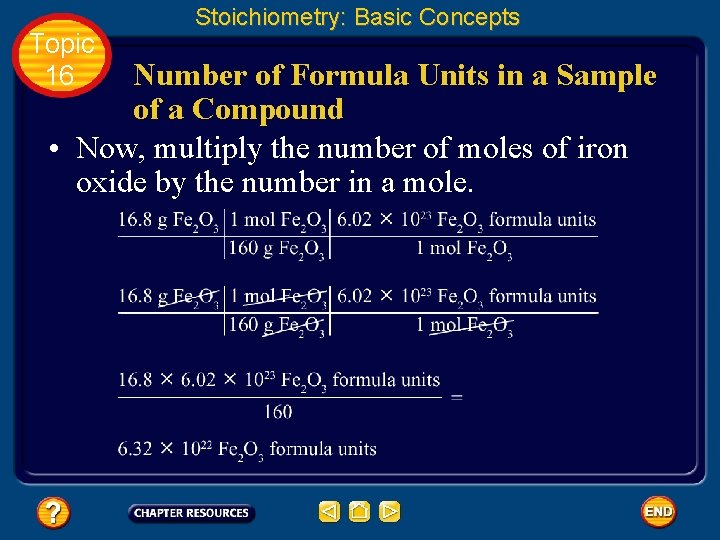Topic 16 Stoichiometry: Basic Concepts Number of Formula Units in a Sample of a Compound • Now, multiply the number of moles of iron oxide by the number in a mole.Topic 16 Stoichiometry: Basic Concepts Mass of a Number of Moles of a Compound • What mass of water must be weighed to obtain 7. 50 mol of H 2 O? • The molar mass of water is obtained from its molecular mass. • The molar mass of water is 18. 0 g/mol.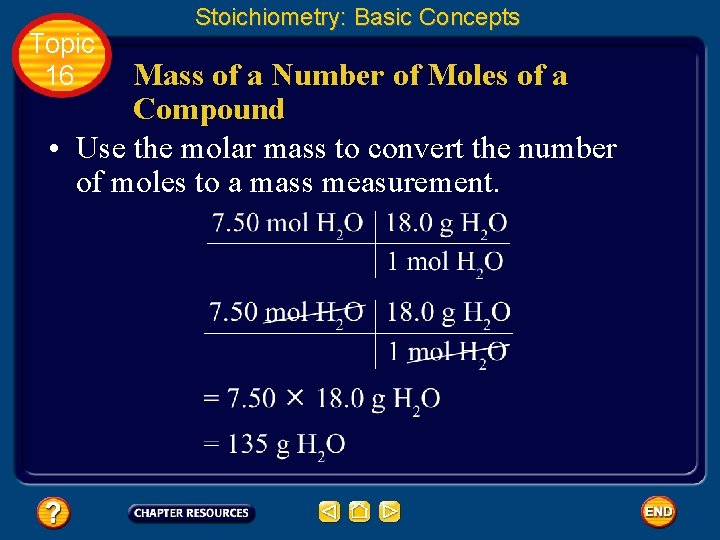Topic 16 Stoichiometry: Basic Concepts Mass of a Number of Moles of a Compound • Use the molar mass to convert the number of moles to a mass measurement.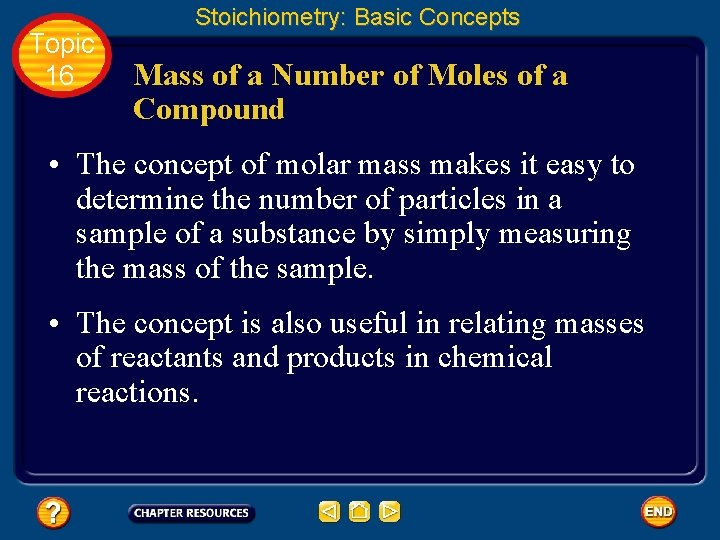Topic 16 Stoichiometry: Basic Concepts Mass of a Number of Moles of a Compound • The concept of molar mass makes it easy to determine the number of particles in a sample of a substance by simply measuring the mass of the sample. • The concept is also useful in relating masses of reactants and products in chemical reactions.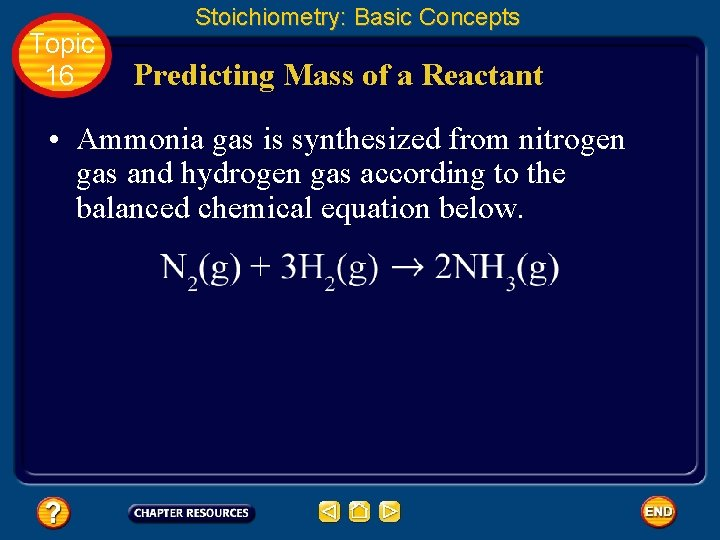Topic 16 Stoichiometry: Basic Concepts Predicting Mass of a Reactant • Ammonia gas is synthesized from nitrogen gas and hydrogen gas according to the balanced chemical equation below.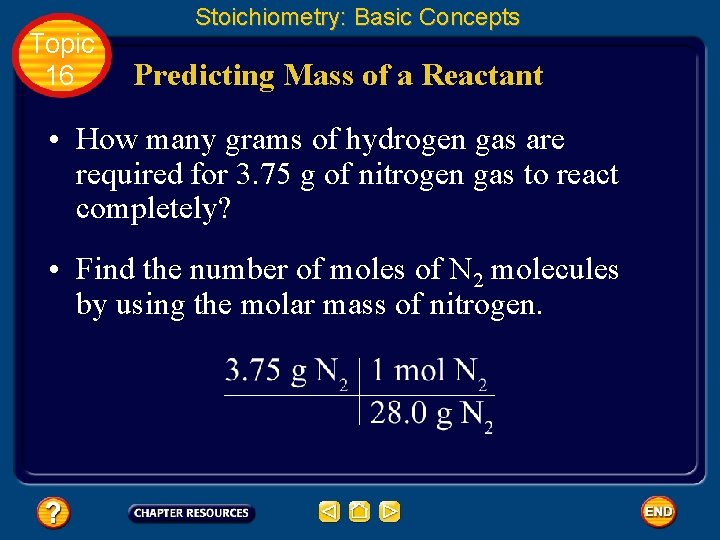Topic 16 Stoichiometry: Basic Concepts Predicting Mass of a Reactant • How many grams of hydrogen gas are required for 3. 75 g of nitrogen gas to react completely? • Find the number of moles of N 2 molecules by using the molar mass of nitrogen.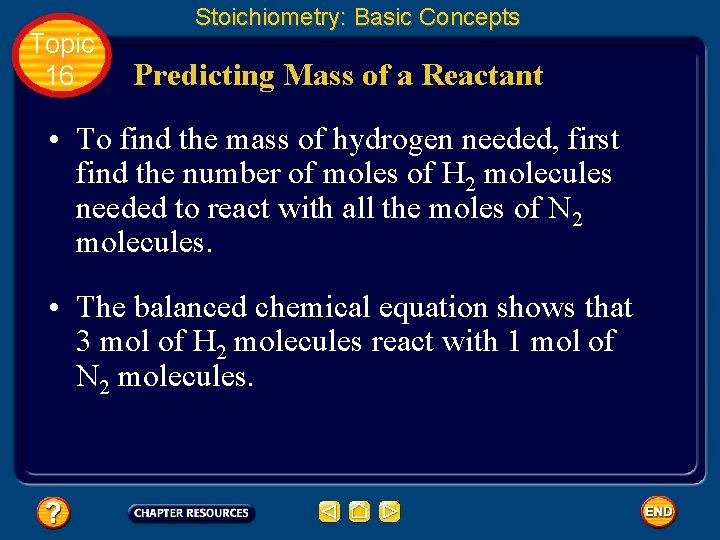Topic 16 Stoichiometry: Basic Concepts Predicting Mass of a Reactant • To find the mass of hydrogen needed, first find the number of moles of H 2 molecules needed to react with all the moles of N 2 molecules. • The balanced chemical equation shows that 3 mol of H 2 molecules react with 1 mol of N 2 molecules.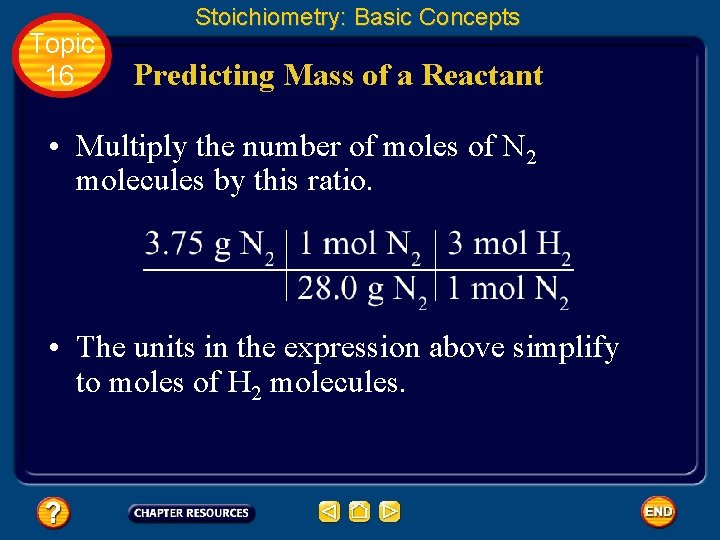Topic 16 Stoichiometry: Basic Concepts Predicting Mass of a Reactant • Multiply the number of moles of N 2 molecules by this ratio. • The units in the expression above simplify to moles of H 2 molecules.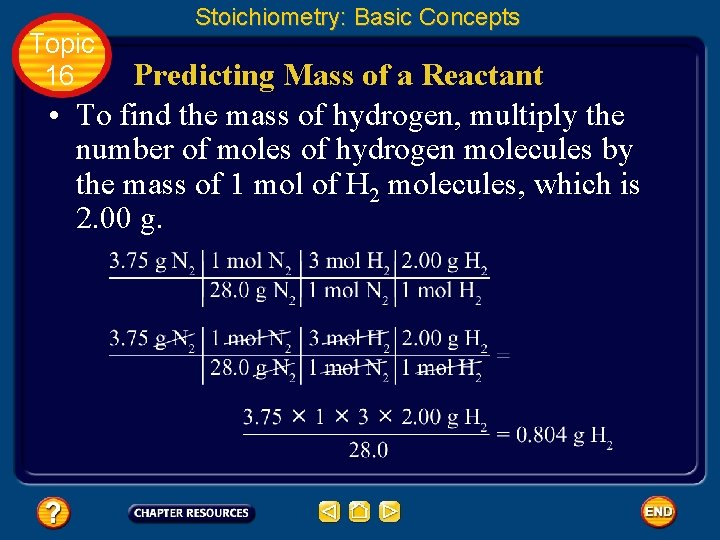Topic 16 Stoichiometry: Basic Concepts Predicting Mass of a Reactant • To find the mass of hydrogen, multiply the number of moles of hydrogen molecules by the mass of 1 mol of H 2 molecules, which is 2. 00 g.Topic 16 Stoichiometry: Basic Concepts Predicting Mass of a Product • What mass of ammonia is formed when 3. 75 g of nitrogen gas react with hydrogen gas according to the balanced chemical equation below? • The amount of ammonia formed depends upon the number of nitrogen molecules present and the mole ratio of nitrogen and ammonia in the balanced chemical equation.Topic 16 Stoichiometry: Basic Concepts Predicting Mass of a Product • The number of moles of nitrogen molecules is given by the expression below.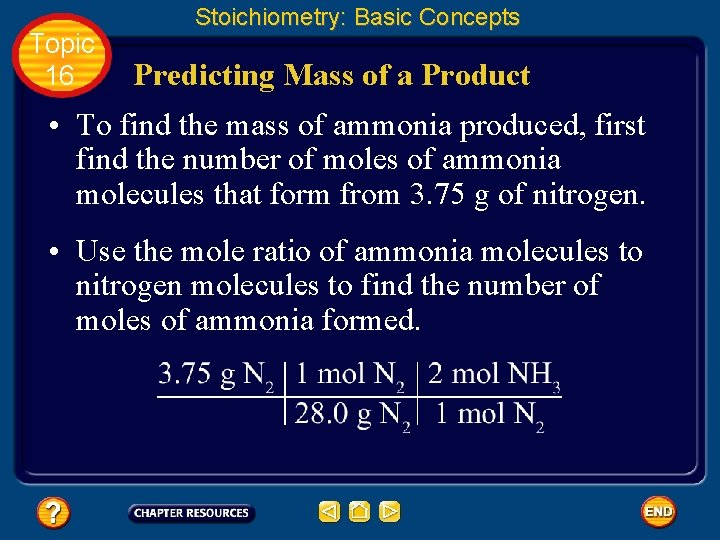Topic 16 Stoichiometry: Basic Concepts Predicting Mass of a Product • To find the mass of ammonia produced, first find the number of moles of ammonia molecules that form from 3. 75 g of nitrogen. • Use the mole ratio of ammonia molecules to nitrogen molecules to find the number of moles of ammonia formed.Topic 16 Stoichiometry: Basic Concepts Predicting Mass of a Product • Use the molar mass of ammonia, 17. 0 g, to find the mass of ammonia formed.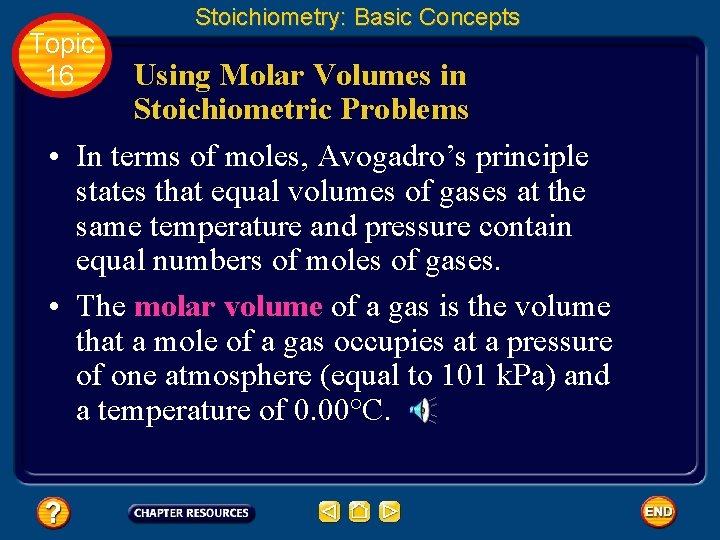Topic 16 Stoichiometry: Basic Concepts Using Molar Volumes in Stoichiometric Problems • In terms of moles, Avogadro’s principle states that equal volumes of gases at the same temperature and pressure contain equal numbers of moles of gases. • The molar volume of a gas is the volume that a mole of a gas occupies at a pressure of one atmosphere (equal to 101 k. Pa) and a temperature of 0. 00°C.Topic 16 Stoichiometry: Basic Concepts Using Molar Volumes in Stoichiometric Problems • Under these conditions of STP, the volume of 1 mol of any gas is 22. 4 L. • Like the molar mass, the molar volume is used in stoichiometric calculations.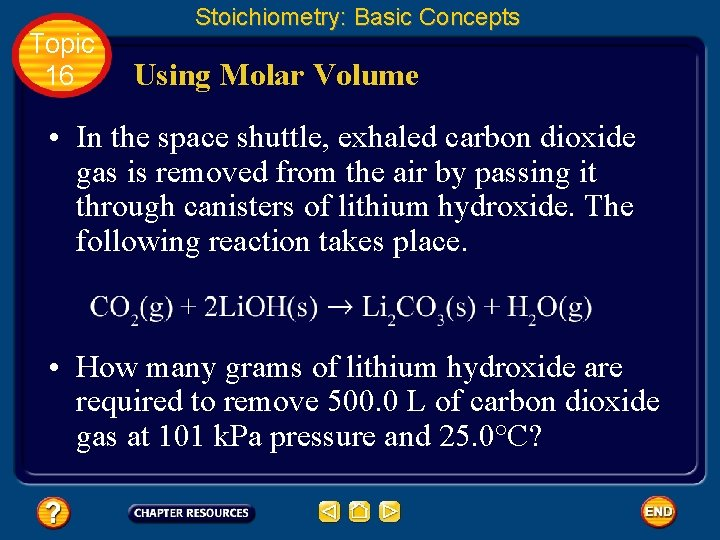Topic 16 Stoichiometry: Basic Concepts Using Molar Volume • In the space shuttle, exhaled carbon dioxide gas is removed from the air by passing it through canisters of lithium hydroxide. The following reaction takes place. • How many grams of lithium hydroxide are required to remove 500. 0 L of carbon dioxide gas at 101 k. Pa pressure and 25. 0°C?Topic 16 Stoichiometry: Basic Concepts Using Molar Volume • The volume of gas at 25°C must be converted to a volume at STP. • Now, find the number of moles of CO 2 gas as below.Topic 16 Stoichiometry: Basic Concepts Using Molar Volume • The chemical equation shows that the ratio of moles of Li. OH to CO 2 is 2 to 1. • Therefore, the number of moles of lithium hydroxide is given by the expression below. • To convert the number of moles of Li. OH to mass, use its molar mass, 23. 9 g/mol.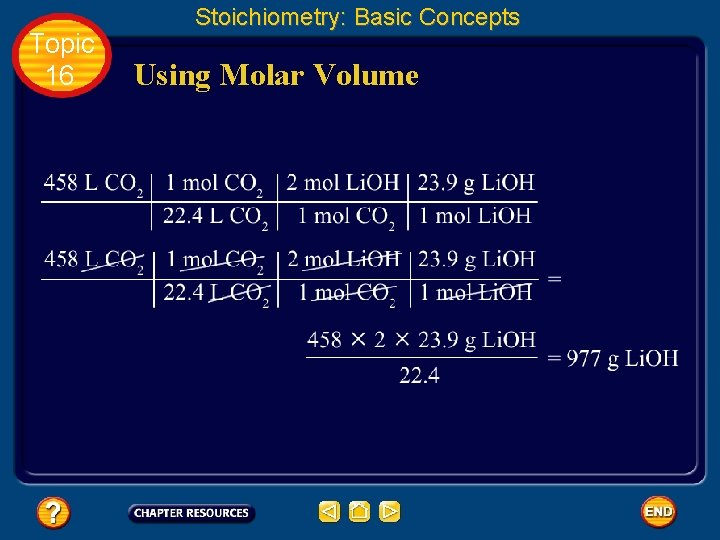Topic 16 Stoichiometry: Basic Concepts Using Molar Volume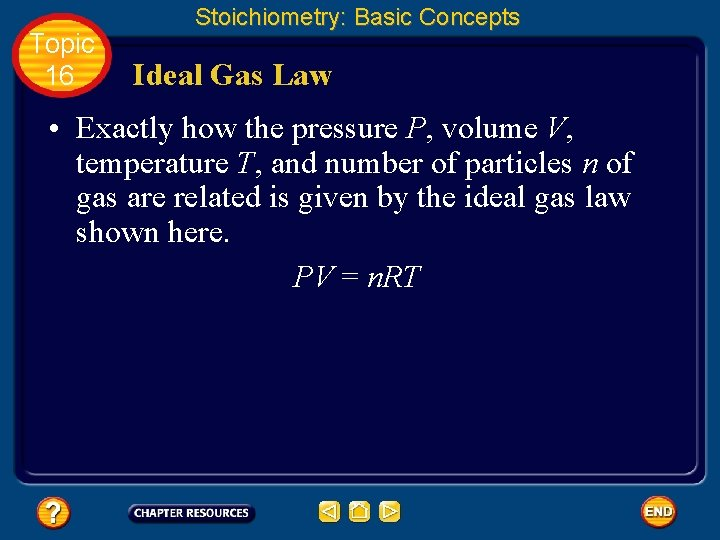Topic 16 Stoichiometry: Basic Concepts Ideal Gas Law • Exactly how the pressure P, volume V, temperature T, and number of particles n of gas are related is given by the ideal gas law shown here. PV = n. RT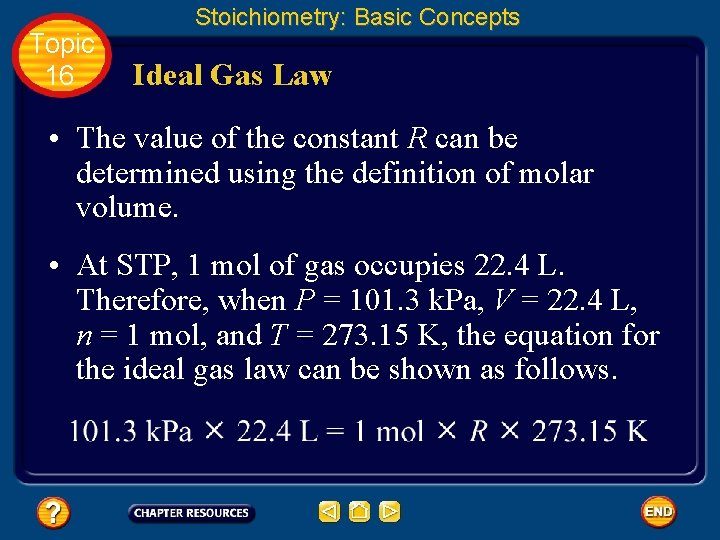Topic 16 Stoichiometry: Basic Concepts Ideal Gas Law • The value of the constant R can be determined using the definition of molar volume. • At STP, 1 mol of gas occupies 22. 4 L. Therefore, when P = 101. 3 k. Pa, V = 22. 4 L, n = 1 mol, and T = 273. 15 K, the equation for the ideal gas law can be shown as follows.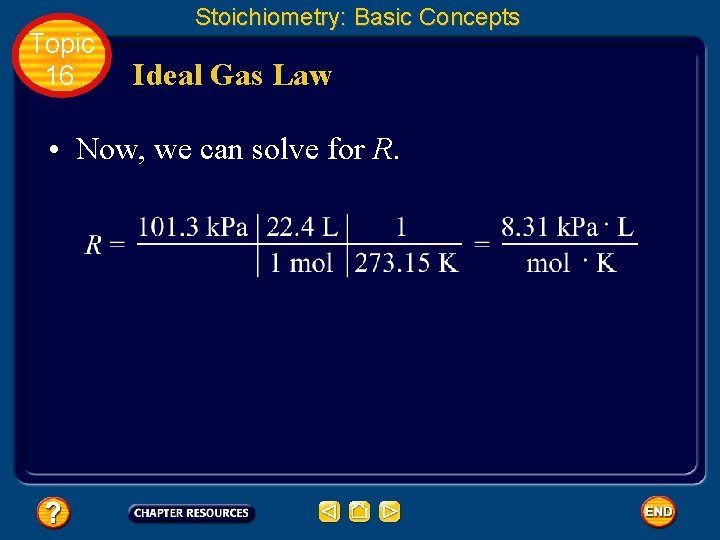Topic 16 Stoichiometry: Basic Concepts Ideal Gas Law • Now, we can solve for R.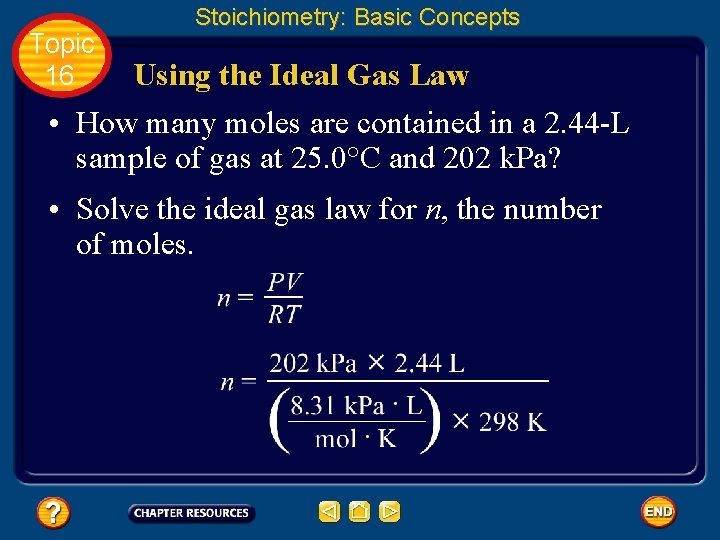Topic 16 Stoichiometry: Basic Concepts Using the Ideal Gas Law • How many moles are contained in a 2. 44 -L sample of gas at 25. 0°C and 202 k. Pa? • Solve the ideal gas law for n, the number of moles.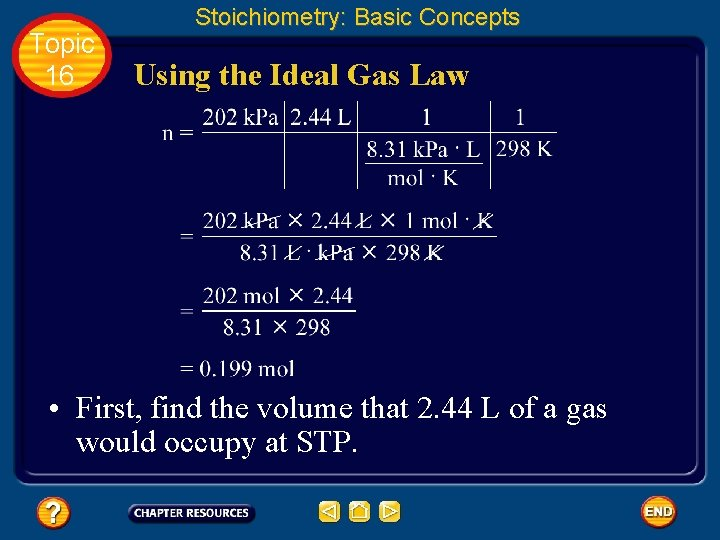Topic 16 Stoichiometry: Basic Concepts Using the Ideal Gas Law • First, find the volume that 2. 44 L of a gas would occupy at STP.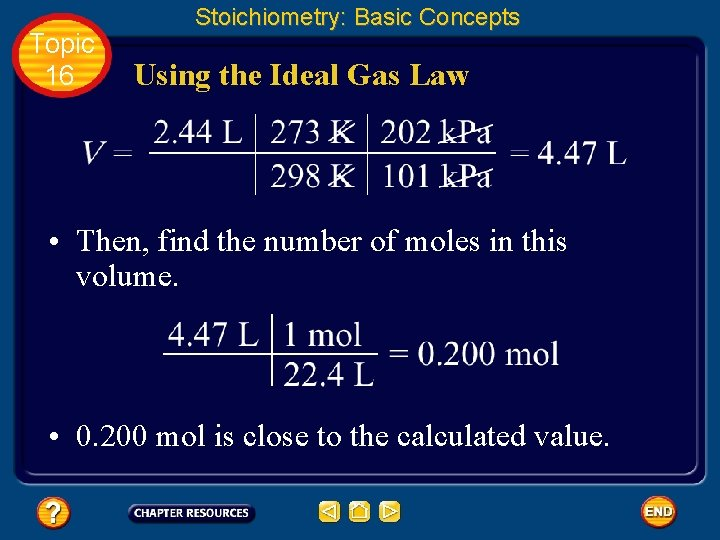Topic 16 Stoichiometry: Basic Concepts Using the Ideal Gas Law • Then, find the number of moles in this volume. • 0. 200 mol is close to the calculated value.Topic 16 Stoichiometry: Basic Concepts Determining Mass Percents • The formula for geraniol (the main compound that gives a rose its scent) is C 10 H 18 O.Topic 16 Stoichiometry: Basic Concepts Determining Mass Percents • The formula shows that geraniol is comprised of carbon, hydrogen, and oxygen. • Because all these elements are nonmetals, geraniol is probably covalent and comprised of molecules.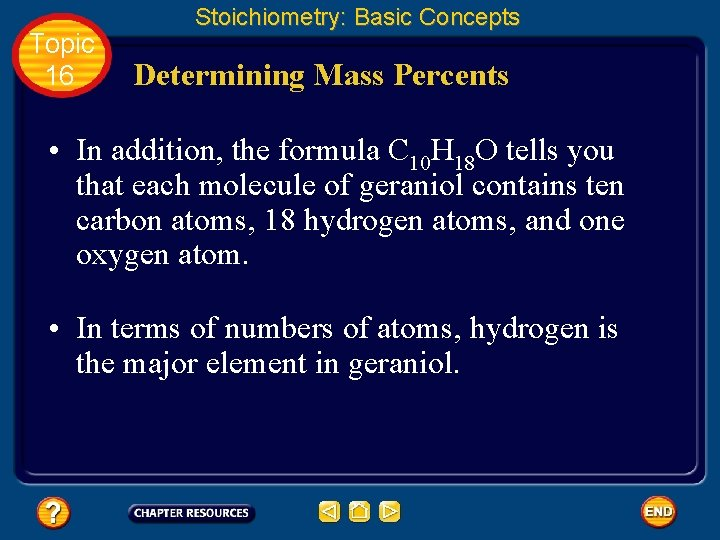Topic 16 Stoichiometry: Basic Concepts Determining Mass Percents • In addition, the formula C 10 H 18 O tells you that each molecule of geraniol contains ten carbon atoms, 18 hydrogen atoms, and one oxygen atom. • In terms of numbers of atoms, hydrogen is the major element in geraniol.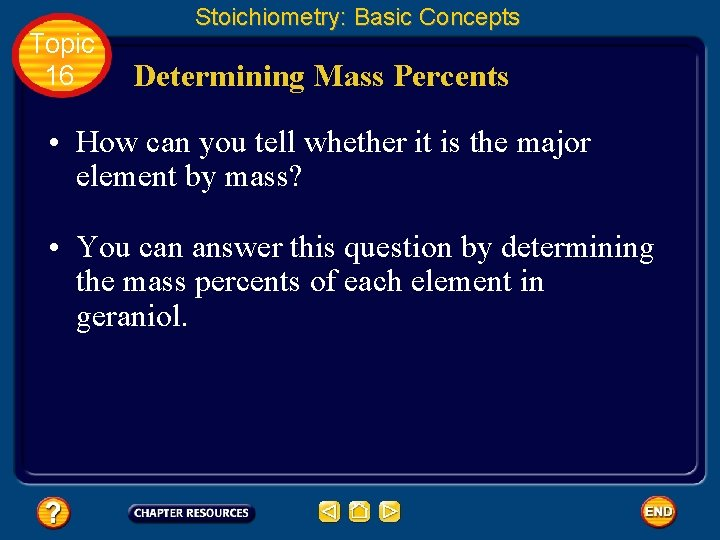Topic 16 Stoichiometry: Basic Concepts Determining Mass Percents • How can you tell whether it is the major element by mass? • You can answer this question by determining the mass percents of each element in geraniol.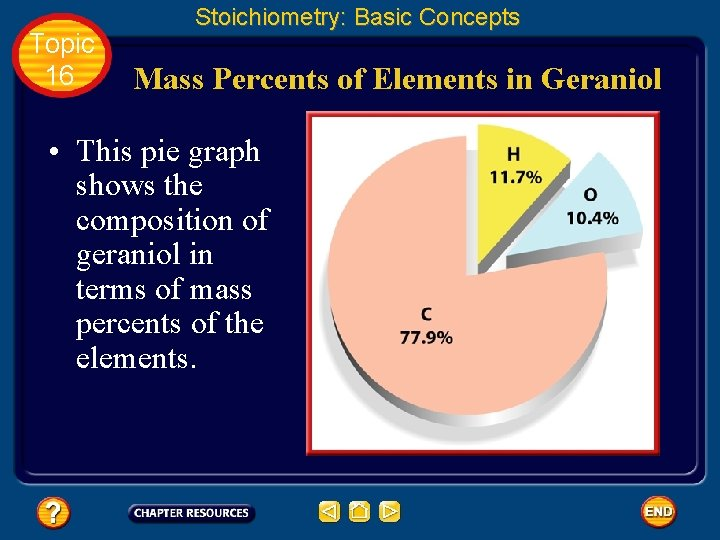Topic 16 Stoichiometry: Basic Concepts Mass Percents of Elements in Geraniol • This pie graph shows the composition of geraniol in terms of mass percents of the elements.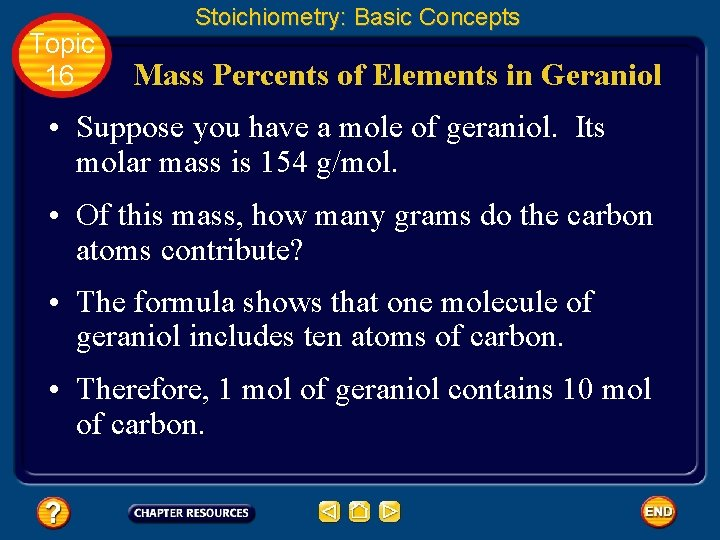Topic 16 Stoichiometry: Basic Concepts Mass Percents of Elements in Geraniol • Suppose you have a mole of geraniol. Its molar mass is 154 g/mol. • Of this mass, how many grams do the carbon atoms contribute? • The formula shows that one molecule of geraniol includes ten atoms of carbon. • Therefore, 1 mol of geraniol contains 10 mol of carbon.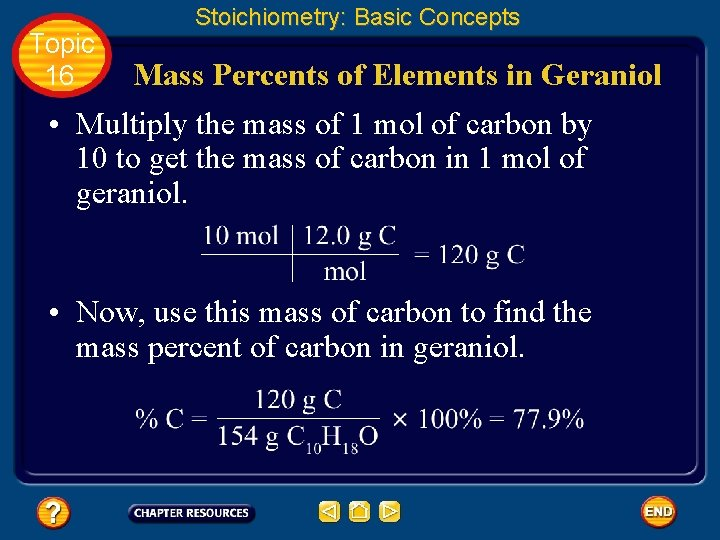Topic 16 Stoichiometry: Basic Concepts Mass Percents of Elements in Geraniol • Multiply the mass of 1 mol of carbon by 10 to get the mass of carbon in 1 mol of geraniol. • Now, use this mass of carbon to find the mass percent of carbon in geraniol.Topic 16 Stoichiometry: Basic Concepts Mass Percents of Elements in Geraniol • The mass percents of the other elements are calculated below in a similar fashion. • Mass of hydrogen in 1 mol geraniol:Topic 16 Stoichiometry: Basic Concepts Mass Percents of Elements in Geraniol • Mass of oxygen in 1 mol geraniol: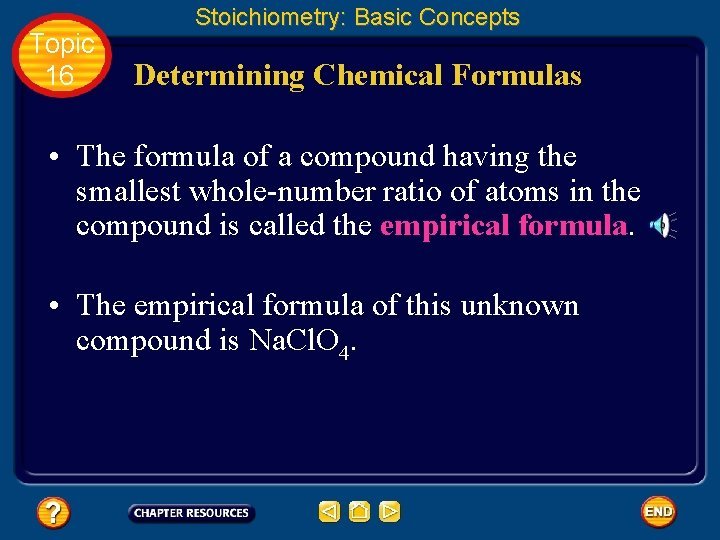Topic 16 Stoichiometry: Basic Concepts Determining Chemical Formulas • The formula of a compound having the smallest whole-number ratio of atoms in the compound is called the empirical formula. • The empirical formula of this unknown compound is Na. Cl. O 4.Topic 16 Basic Assessment Questions Question 1 Determine the number of atoms in 45. 6 g gold, Au.Topic 16 Basic Assessment Questions Answer 1. 39 x 1023 Au atoms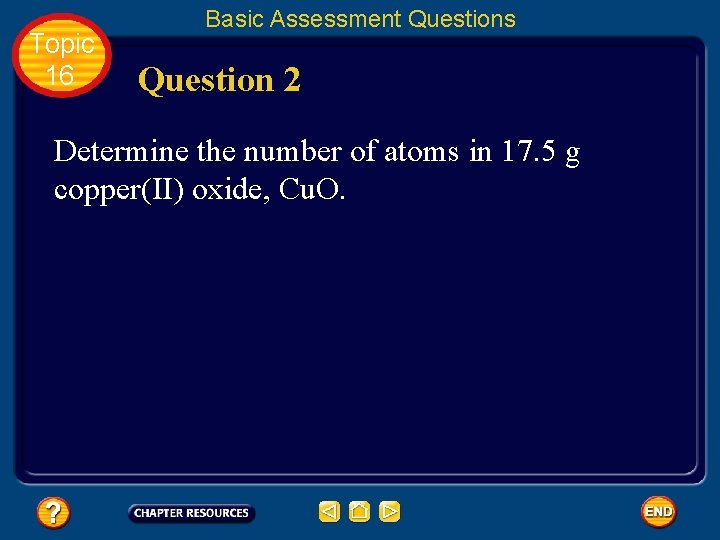Topic 16 Basic Assessment Questions Question 2 Determine the number of atoms in 17. 5 g copper(II) oxide, Cu. O.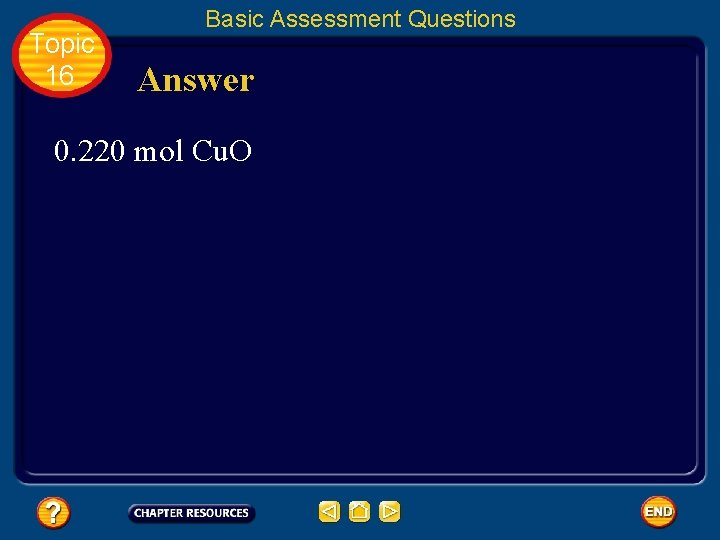Topic 16 Basic Assessment Questions Answer 0. 220 mol Cu. OTopic 16 Basic Assessment Questions Question 3 Determine the mass of 1. 25 mol aspirin C 9 H 8 O 4.Topic 16 Basic Assessment Questions Answer 225 g C 9 H 8 O 4Topic 16 Basic Assessment Questions Question 4 What mass of sulfur must burn to produce 3. 42 L of SO 2 at 273°C and 101 k. Pa? The reaction is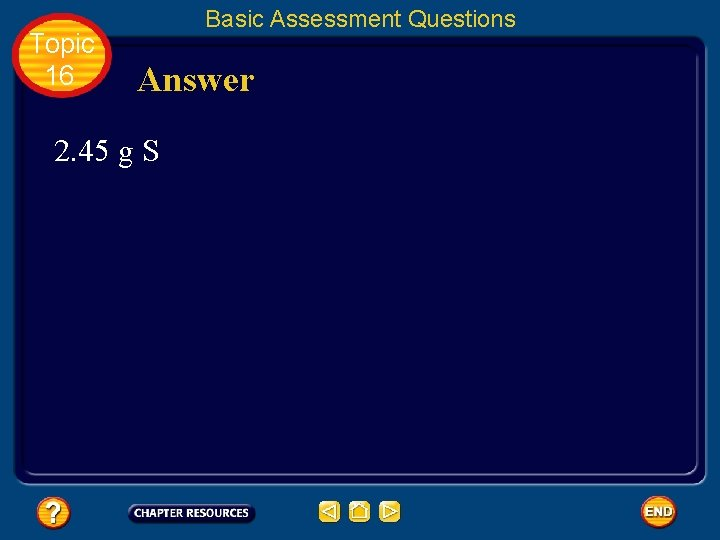Topic 16 Basic Assessment Questions Answer 2. 45 g S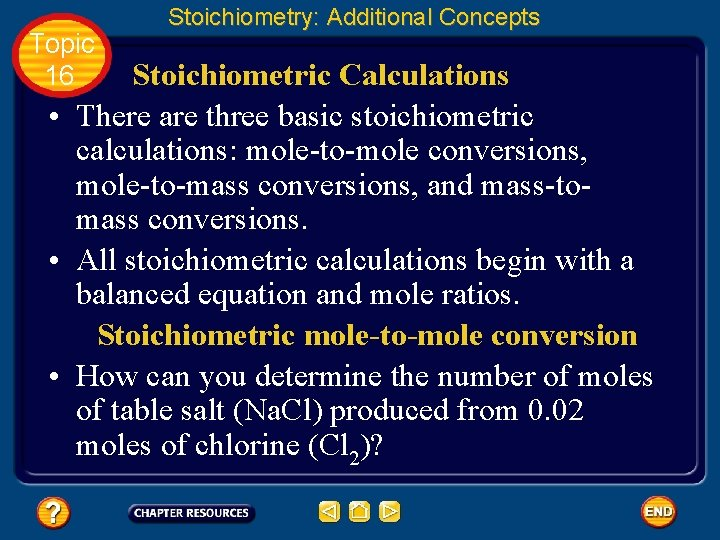Topic 16 Stoichiometry: Additional Concepts Stoichiometric Calculations • There are three basic stoichiometric calculations: mole-to-mole conversions, mole-to-mass conversions, and mass-tomass conversions. • All stoichiometric calculations begin with a balanced equation and mole ratios. Stoichiometric mole-to-mole conversion • How can you determine the number of moles of table salt (Na. Cl) produced from 0. 02 moles of chlorine (Cl 2)?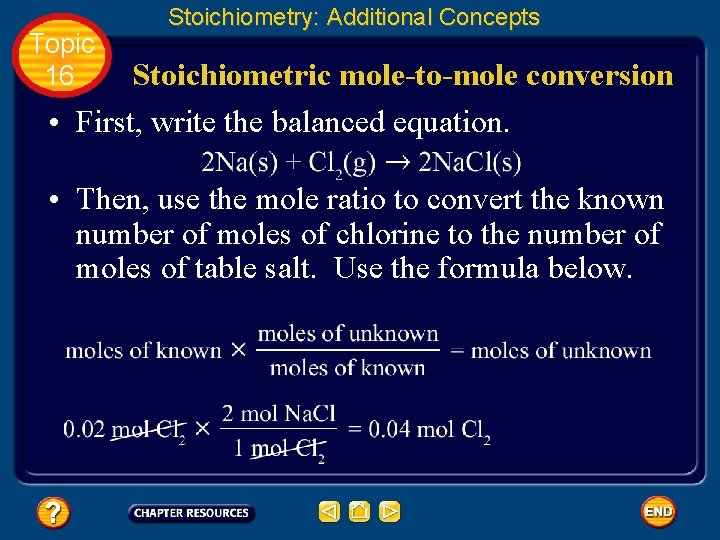Topic 16 Stoichiometry: Additional Concepts Stoichiometric mole-to-mole conversion • First, write the balanced equation. • Then, use the mole ratio to convert the known number of moles of chlorine to the number of moles of table salt. Use the formula below.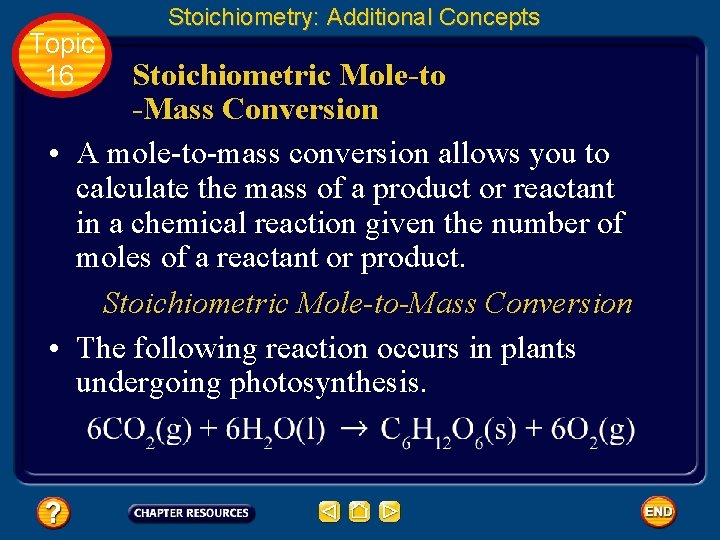Topic 16 Stoichiometry: Additional Concepts Stoichiometric Mole-to -Mass Conversion • A mole-to-mass conversion allows you to calculate the mass of a product or reactant in a chemical reaction given the number of moles of a reactant or product. Stoichiometric Mole-to-Mass Conversion • The following reaction occurs in plants undergoing photosynthesis.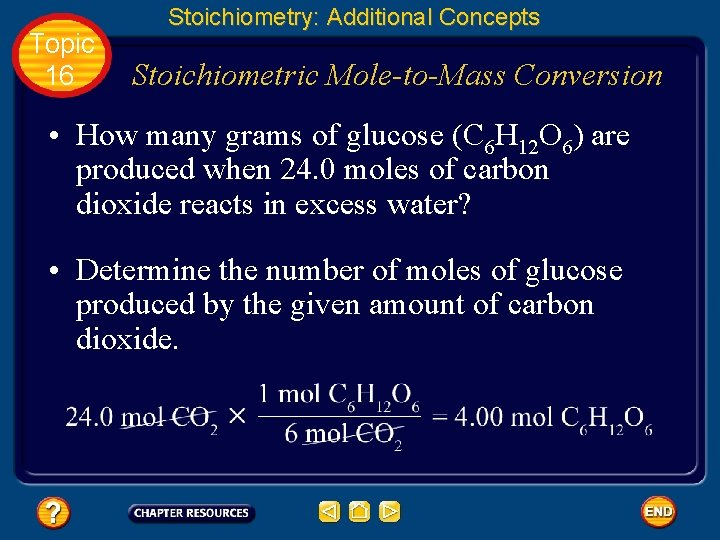Topic 16 Stoichiometry: Additional Concepts Stoichiometric Mole-to-Mass Conversion • How many grams of glucose (C 6 H 12 O 6) are produced when 24. 0 moles of carbon dioxide reacts in excess water? • Determine the number of moles of glucose produced by the given amount of carbon dioxide.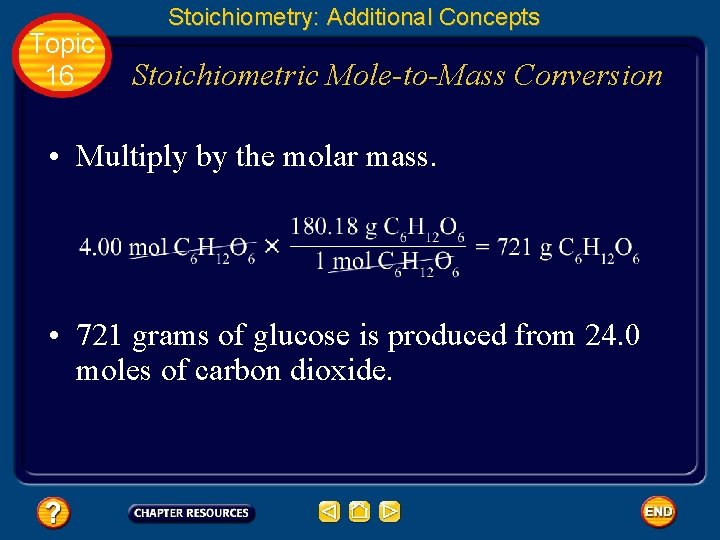Topic 16 Stoichiometry: Additional Concepts Stoichiometric Mole-to-Mass Conversion • Multiply by the molar mass. • 721 grams of glucose is produced from 24. 0 moles of carbon dioxide.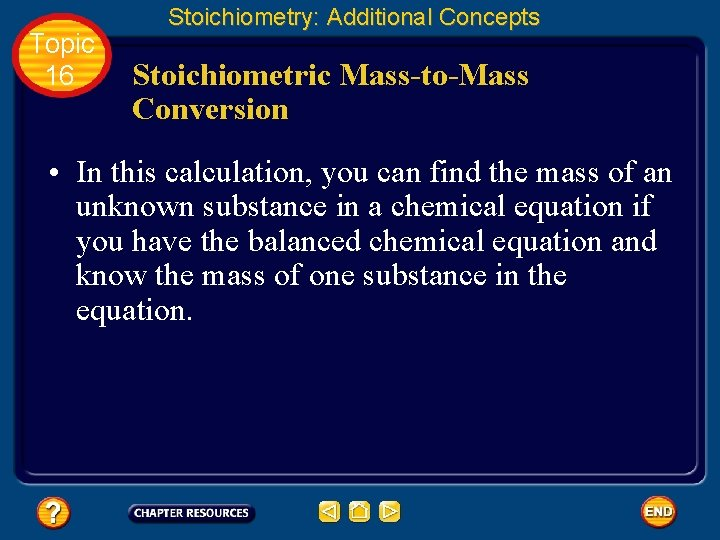Topic 16 Stoichiometry: Additional Concepts Stoichiometric Mass-to-Mass Conversion • In this calculation, you can find the mass of an unknown substance in a chemical equation if you have the balanced chemical equation and know the mass of one substance in the equation.Topic 16 Stoichiometry: Additional Concepts Stoichiometric Mass-to-Mass Conversion • How many grams of sodium hydroxide (Na. OH) are needed to completely react with 50. 0 grams of sulfuric acid (H 2 SO 4) to form sodium sulfate (Na 2 SO 4) and water? • Write the balanced equation.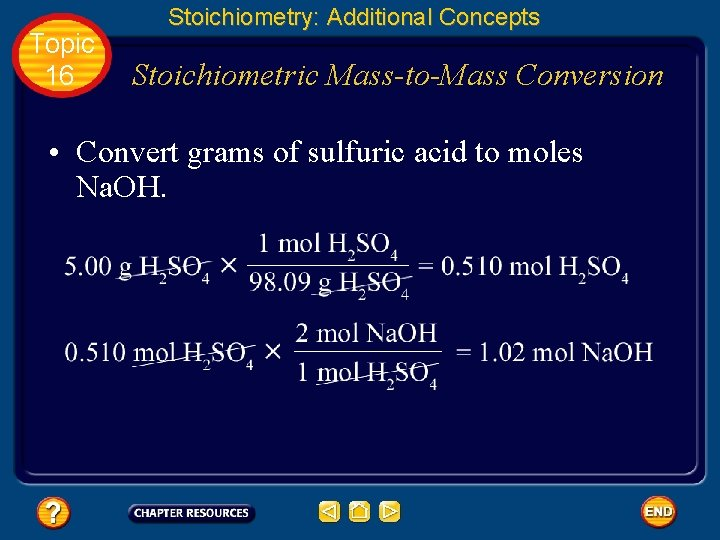Topic 16 Stoichiometry: Additional Concepts Stoichiometric Mass-to-Mass Conversion • Convert grams of sulfuric acid to moles Na. OH.Topic 16 Stoichiometry: Additional Concepts Stoichiometric Mass-to-Mass Conversion • Calculate the mass of Na. OH needed. • 50. 0 grams of H 2 SO 4 reacts completely with 40. 8 grams of Na. OH.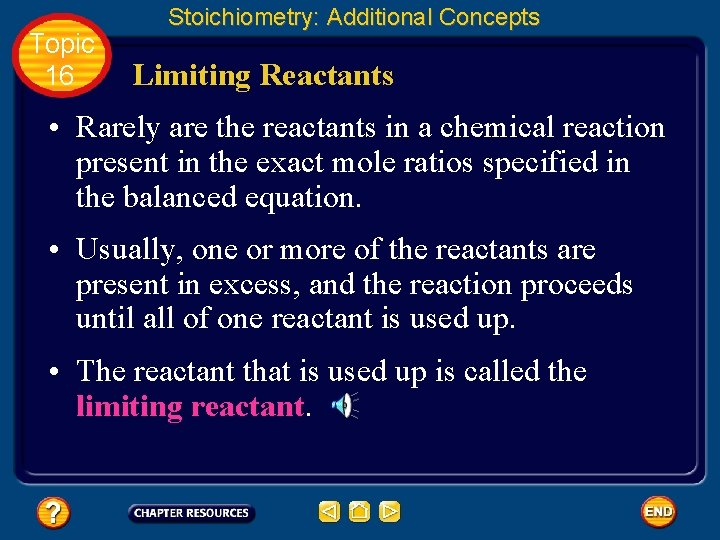Topic 16 Stoichiometry: Additional Concepts Limiting Reactants • Rarely are the reactants in a chemical reaction present in the exact mole ratios specified in the balanced equation. • Usually, one or more of the reactants are present in excess, and the reaction proceeds until all of one reactant is used up. • The reactant that is used up is called the limiting reactant.Topic 16 • • Stoichiometry: Additional Concepts Limiting Reactants The limiting reactant limits the reaction and, thus, determines how much of the product forms. The left-over reactants are called excess reactants. How can you determine which reactant in a chemical reaction is limited? First, find the number of moles of each reactant by multiplying the given mass of each reactant by the inverse of the molar mass.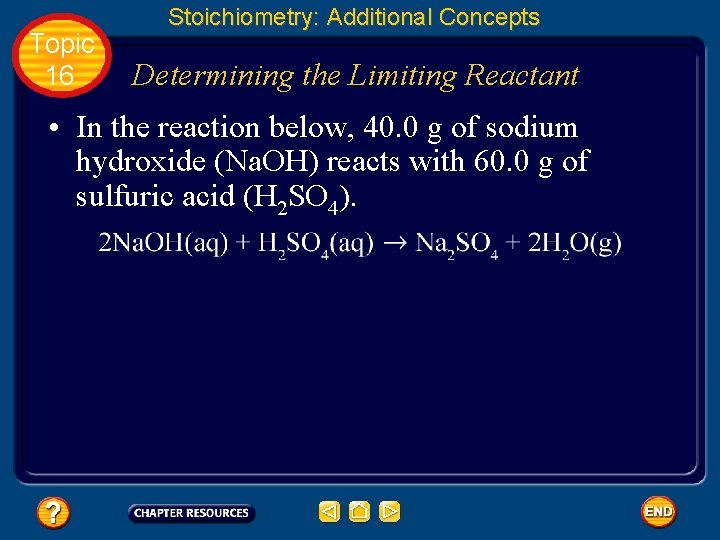Topic 16 Stoichiometry: Additional Concepts Determining the Limiting Reactant • In the reaction below, 40. 0 g of sodium hydroxide (Na. OH) reacts with 60. 0 g of sulfuric acid (H 2 SO 4).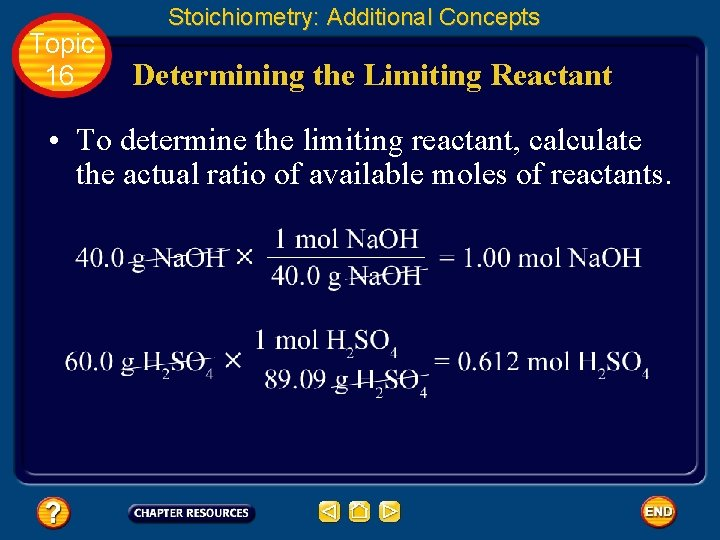Topic 16 Stoichiometry: Additional Concepts Determining the Limiting Reactant • To determine the limiting reactant, calculate the actual ratio of available moles of reactants.Topic 16 Stoichiometry: Additional Concepts Determining the Limiting Reactant • So, is available. Compare this ratio with the mole ratio from the balanced equation: , or • You can see that when 0. 5 mol H 2 SO 4 has reacted, all of the 1. 00 mol of Na. OH would be used up. • Some H 2 SO 4 would remain unreacted. Thus, Na. OH is the limiting reactant.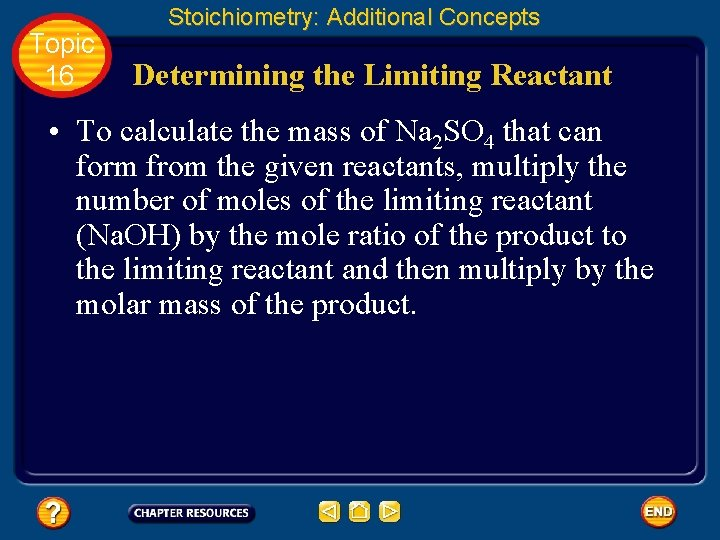Topic 16 Stoichiometry: Additional Concepts Determining the Limiting Reactant • To calculate the mass of Na 2 SO 4 that can form from the given reactants, multiply the number of moles of the limiting reactant (Na. OH) by the mole ratio of the product to the limiting reactant and then multiply by the molar mass of the product.Topic 16 Stoichiometry: Additional Concepts Determining the Limiting Reactant • 71. 0 g of Na 2 SO 4 can form from the given amounts of the reactants.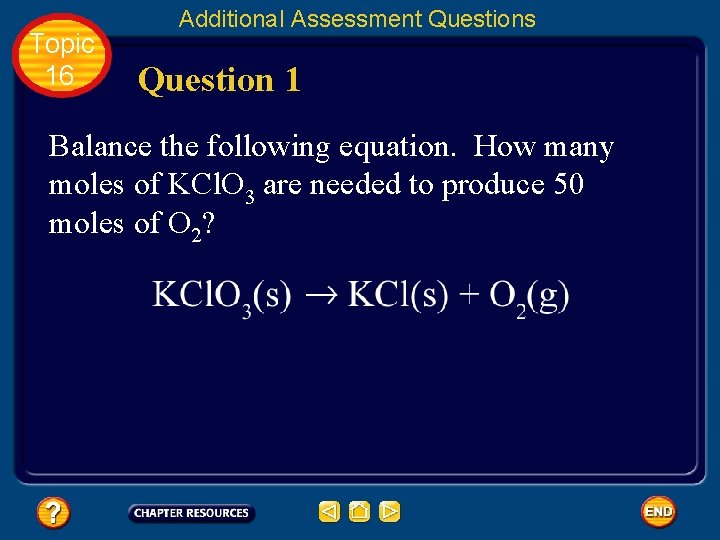Topic 16 Additional Assessment Questions Question 1 Balance the following equation. How many moles of KCl. O 3 are needed to produce 50 moles of O 2?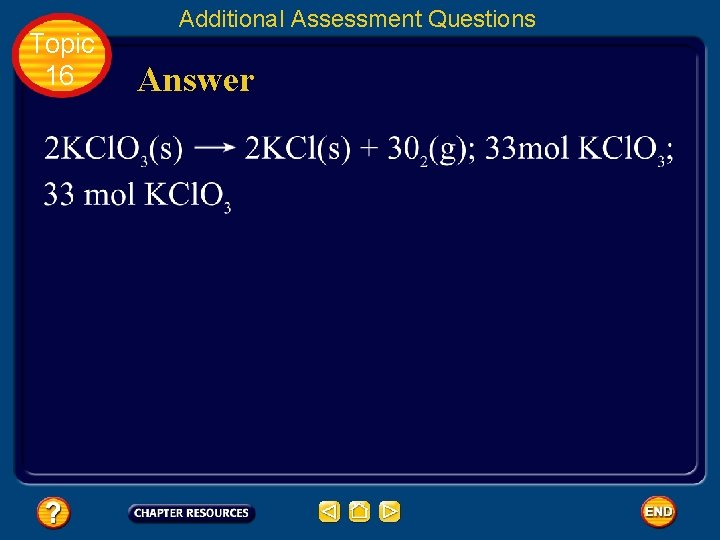Topic 16 Additional Assessment Questions Question 2 Calculate the mass of sodium chloride (Na. Cl) produced when 5. 50 moles of sodium reacts in excess chlorine gas.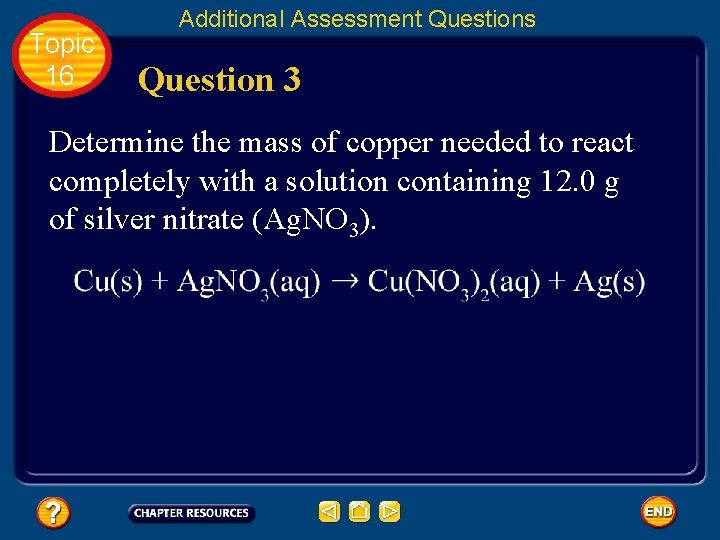Topic 16 Additional Assessment Questions Question 3 Determine the mass of copper needed to react completely with a solution containing 12. 0 g of silver nitrate (Ag. NO 3).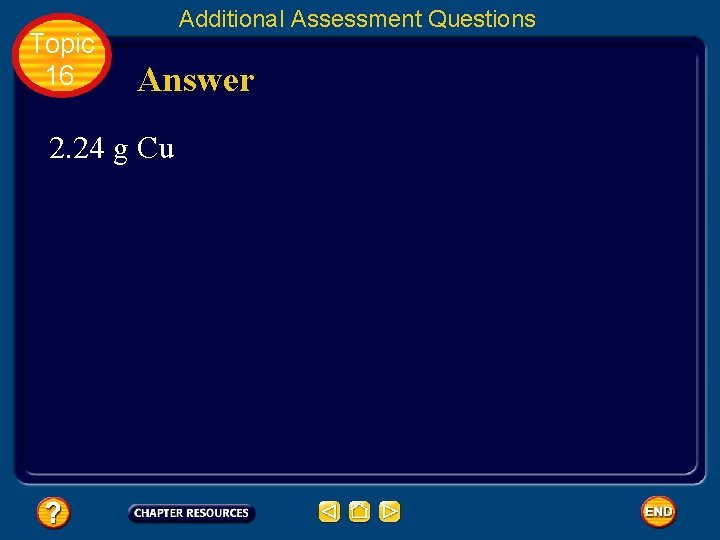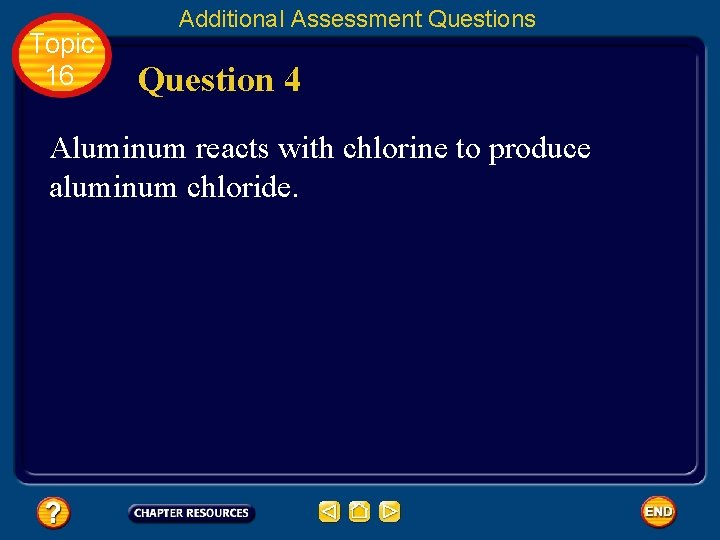Topic 16 Additional Assessment Questions Question 4 Aluminum reacts with chlorine to produce aluminum chloride.Topic 16 Additional Assessment Questions Question 4 a Balance the equation. Answer 4 a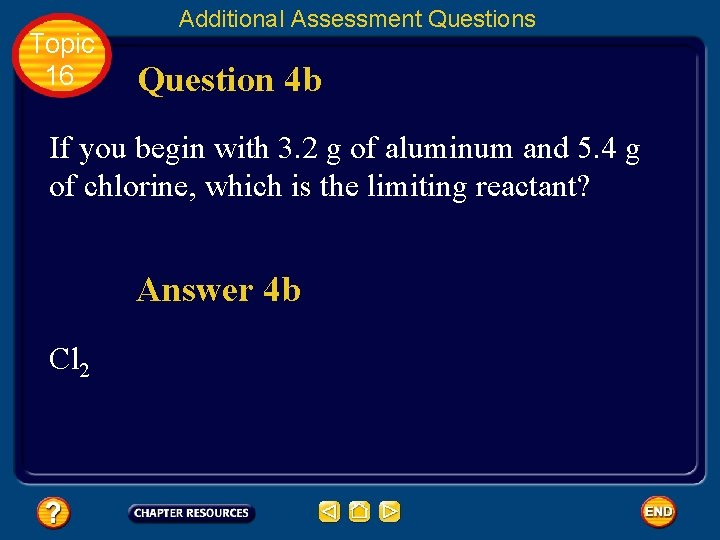Topic 16 Additional Assessment Questions Question 4 b If you begin with 3. 2 g of aluminum and 5. 4 g of chlorine, which is the limiting reactant? Answer 4 b Cl 2Help To advance to the next item or next page click on any of the following keys: mouse, space bar, enter, down or forward arrow. Click on this icon to return to the table of contents Click on this icon to return to the previous slide Click on this icon to move to the next slide Click on this icon to open the resources file. Click on this icon to go to the end of the presentation.End of Topic Summary File